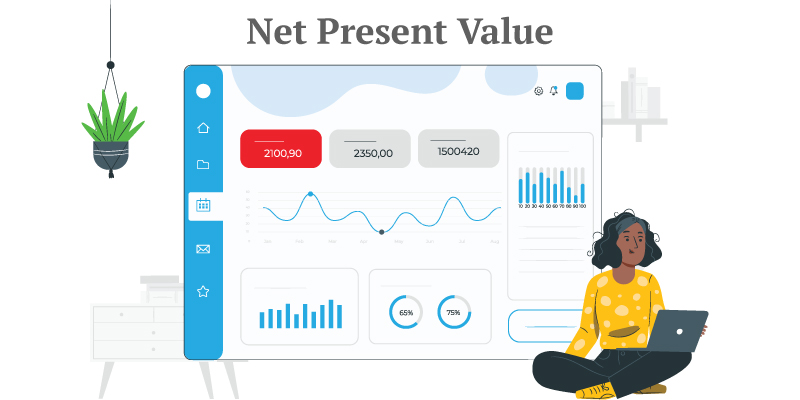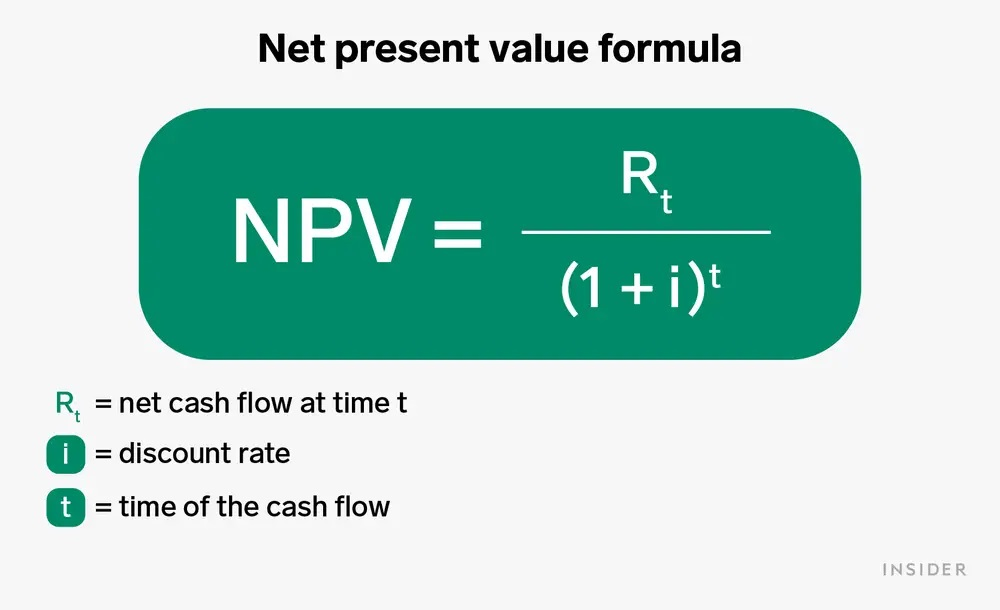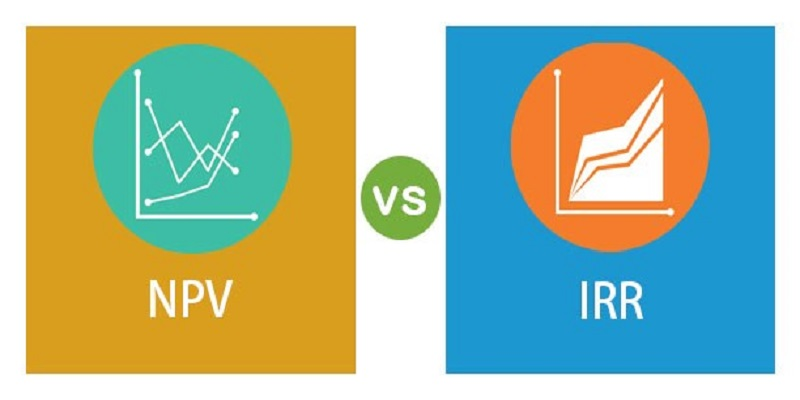Spring Promo on now! For a limited time, submit a FREE application (\$50 value)!# Net Present Value - A Need To Know in Property Investing

### Alcove Team·5/15/23Investing in property is one of the most popular methods for generating long-term income. As an investor, it is important to understand key concepts that can help you make better investment decisions. One of these concepts is net present value (NPV), which is a measure of the present value of future cash flows. In this blog post, we will discuss what NPV is, how it is calculated, and its importance in property investing.

### What is Net Present Value (NPV)Net present value (NPV) is the difference between the present value of cash inflows and the present value of cash outflows. It is used to determine the value of an investment by considering the time value of money. The time value of money refers to the fact that money available at the present time is worth more than the same amount in the future because of its potential earning capacity.

### How is Net Present Value CalculatedCalculating NPV involves the following steps:

1. Estimate the expected cash flows for the investment. This includes all expected income generated from the investment, as well as any expenses related to the investment.

2. Determine the discount rate. This is the rate of return that an investor would require to invest in a similar investment. The discount rate takes into account the risk associated with the investment.

3. Calculate the present value of each cash flow. This involves discounting each cash flow by the discount rate. The present value of each cash flow is calculated by dividing the cash flow by (1 + discount rate) to the power of the number of years into the future the cash flow is expected.

4. Add up the present value of all expected cash inflows and subtract the present value of all expected cash outflows.

The resulting number is the NPV of the investment. If the NPV is positive, the investment is expected to be profitable. If the NPV is negative, the investment is expected to generate a loss.

### Importance of Net Present Value in Property Investing:NPV is an important tool for property investors because it takes into account the time value of money and inflation. Inflation reduces the purchasing power of money over time, and therefore reduces the value of future cash flows. By discounting future cash flows, NPV takes into account the effect of inflation on the investment.

NPV also allows investors to compare different investment opportunities. For example, an investor may have the option to invest in a rental property or a commercial property. By calculating the NPV of each option, the investor can determine which investment is likely to be more profitable.

### NPV vs Internal Rate of Return (IRR):Another important concept in property investing is the internal rate of return (IRR). IRR is the rate of return that makes the NPV of an investment equal to zero. IRR is often used in conjunction with NPV to evaluate investment opportunities.

While NPV measures the absolute value of an investment, IRR measures the relative value of an investment. IRR is useful when comparing investments with different cash flows or different investment periods. However, IRR assumes that all cash flows are reinvested at the IRR, which may not be realistic.

## Conclusion:

Net present value (NPV) is an important concept in property investing. It allows investors to determine the value of an investment by taking into account the time value of money and inflation. By comparing the NPV of different investment opportunities, investors can make better investment decisions. While IRR is another useful tool for evaluating investment opportunities, it should be used in conjunction with NPV to get a comprehensive understanding of the investment's value. As a property investor, understanding NPV can help you make better investment decisions and increase your chances of generating long-term income.